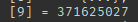# How do u swap values

I want to swap These valueWait, so what are the values you want to swap again?

You could do an extra variable

Example:

a=371625027
b=49348107
c=a
a=b
b=c

You should tell us what you’re trying to do, rather than how you’re trying (and failing) to do it. This is called the XY problem.

That said, it looks like the “how” of what you’re trying to do is flip the key/value pairs in a table where the value becomes the key and vice versa. In theory this isn’t a great idea, because tables have unique indexes while there can be any amount of the same value, so if you tried to flip a table like this:

``````{
 = "hello",
 = "hello"
}
``````

using a function like this:

``````function flipTable(originalTable)
local shallowFlippedTable = {}
for index, value in originalTable do
shallowFlippedTable[value] = index
end
return shallowFlippedTable
end
``````

you’d end up with a table that looks like this:

``````{
["hello"] = 2
}
``````

This topic was automatically closed 14 days after the last reply. New replies are no longer allowed.